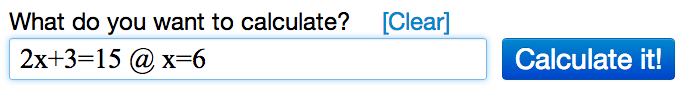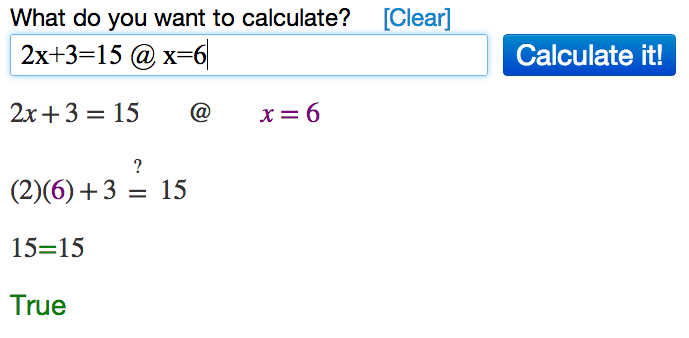# Checking Answers Using Algebra Calculator

Learn how to use the Algebra Calculator to check your answers to algebra problems.

Solve 2x+3=15.

x=6

### How to Check Your Answer with Algebra Calculator

First go to the Algebra Calculator main page.

Type the following:

1. First type the equation 2x+3=15.
2. Then type the @ symbol.
3. Then type x=6.
Try it now: 2x+3=15 @ x=6

### Clickable Demo

Try entering 2x+3=15 @ x=6 into the text box.After you enter the expression, Algebra Calculator will plug x=6 in for the equation 2x+3=15: 2(6)+3 = 15.The calculator prints "True" to let you know that the answer is right.

### More Examples

Here are more examples of how to check your answers with Algebra Calculator. Feel free to try them now.

### Need Help?

Please feel free to Ask MathPapa if you run into problems.